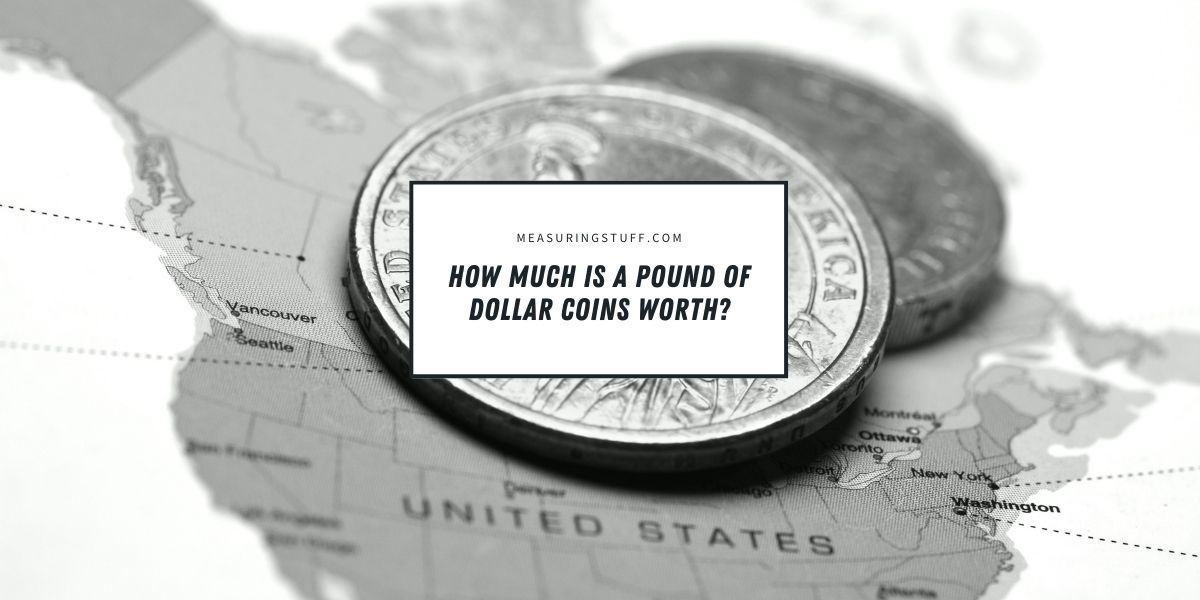# How Much Is A Pound Of Dollar Coins Worth?

Last updated on May 8th, 2023 at 11:12 am

Although dollar coins aren’t as popular as paper dollar bills, they are still in circulation in the United States and are sometimes used as an everyday currency. Like all other United States coins, dollar coins are all the exact same weight when they’re minted, which makes it simple to discover how many are in a given weight and how much that weight is worth.

## How much does a dollar coin weigh?

The first step to finding out how much a pound of dollar coins is worth is finding out how much a single dollar coin weighs. When it’s newly minted, a dollar coin weighs exactly 8.1 grams. Each dollar coin has a diameter of 26.49 millimeters or 1.043 inches and a thickness of 2 millimeters or about 0.0787 inches.

Dollar coins are made of manganese brass, which is 88.5% copper, 6% zinc, 3.5% manganese, and 2% nickel. No other current U.S. coins are made with this particular mixture of metals. Manganese brass has a gold hue.

## How many dollar coins are in 1 pound?

There are a couple of different routes to take to find out how many dollar coins make up 1 pound. First, you can convert grams to ounces and ounces to pounds. There are 28.3495 grams in 1 ounce. Therefore, if we divide 28.3495 by 8.1, we find that you would need about three and a half dollar coins to make up 1 ounce.

There are 16 ounces in 1 pound, so we can multiply 3.5 by 16 to find that there are about 56 dollar coins in 1 pound.

You can use another formula as well, either for simplicity or to check your math. In this case, we can convert grams directly into pounds. There are 453.592 grams in 1 pound. To find how many dollar coins are in a pound using this method, you will need to divide 453.592 by 8.1. The answer is roughly 55.999. Rounded up, we find there are about 56 dollar coins in a pound.

CHECK OUT  How Do You Measure The Thickness Of A Coin?

## How much is a pound of dollar coins worth?

Finding out how much a pound of dollar coins is worth is very simple once you’ve discovered how many coins are in a pound. Since a dollar coin is worth exactly \$1, and there are 56 coins in a pound, 1 pound of dollar coins are worth \$56.

## What is the history of the dollar coin?

The dollar coin was first minted in 1794. From 1794 to 1803, the U.S. dollar coin was considered to be nearly interchangeable with the Spanish dollar, so it was used frequently for purchases overseas or between nations.

Because they were so readily traded to other countries, production of the dollar coin ceased in 1803. The coin reemerged in the 1850s, but there were silver and gold versions, and the silver version was worth slightly more. The gold dollar coin was very small, which made it easy to lose.

In 1878, the government began to mint Morgan dollars. During this time, citizens could purchase silver Morgan dollars with the option to receive a certificate for the value of the dollar. It was at this time that paper money or certificates became more popular than dollar coins.

Because paper bills are easier to carry and much lighter in weight, most people prefer to use them over dollar coins. Dollar coins actually cost less to produce than dollar bills, so the government has repeatedly attempted campaigns to get more people to use them.

This included minting dollar coins with special images on them, including the Sacagawea and Presidential dollar coins. However, when it became clear that they would never gain popularity, the U.S. mint ceased production of dollar coins for general circulation in 2011. Some special dollar coins, however, are still regularly minted.